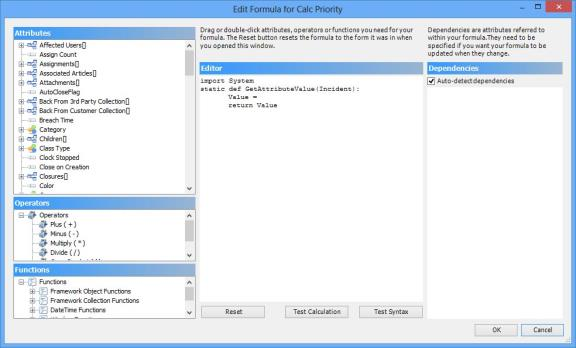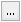## Creating a calculated attribute

Except for calculations as part of a process, you create your calculations in Object Designer by adding a calculation to an attribute.

##### To create a calculated attribute:
1. Start Object Designer, and display the required object.
2. Create a new attribute of the correct Data Type for the calculation you want to create.

For information about creating attributes, see Attributes.

1. In the Properties grid, under Formula, select the required Calculation Type:
None – disables calculations.
Before Save – the value is calculated before the window is saved, or immediately if the Is calculate on change setting is enabled in Window Manager for the dependent attributes used in the calculation. This is the most commonly used calculation type and is typically used if you want to store this value in the database and see the calculated value immediately.
After Read – the value is calculated when it is read from the database and the window is displayed. We recommend that you use this only for date/time calculations that are constantly changing - such as time to breach. In this example, the data displayed by the calculation is correct when the window first appears.

You cannot sort by attributes that are calculated by an After Read calculation.

Scheduled – the value is calculated periodically as defined in Schedule Manager. This is particularly useful for calculating values that you want to report on at predefined intervals, such as asset depreciation values.
Window Calculation – used to dynamically set whether or not fields on a window in Web Access or Ivanti Workspaces are mandatory, read-only, or hidden, based on values selected elsewhere on the window.
The Edit Formula dialog appears.To redisplay the Edit Formula dialog at a later date, clickalongside Calculation Formula in the property grid.

The Dependencies panel appears only for Before Save and Window Calculation calculation types.

1. Complete the calculation, then click OK.
The Edit Formula dialog closes.

For information about creating the calculation, see Using the calculation editor.